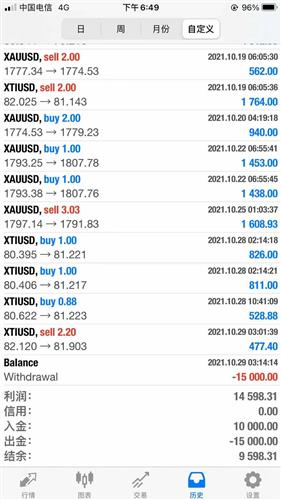1.发行价格

N:=5;
t1:=count(c>o,N)=N;
t2:=count(EVERY(v>ref(v,1) and (c/ref(c,1)-1)*100>10,2),N)>0;
t3:=c<o;
XG:barslast(ref(t1 and t2,1) and t3);张爱玲